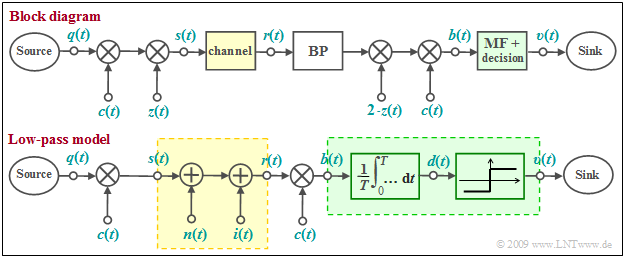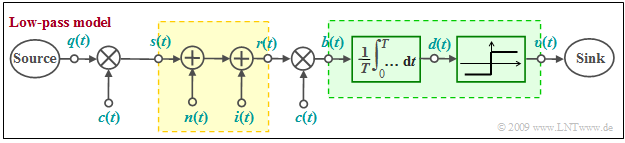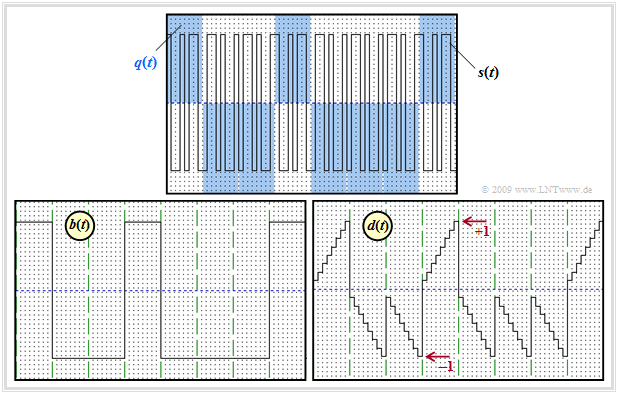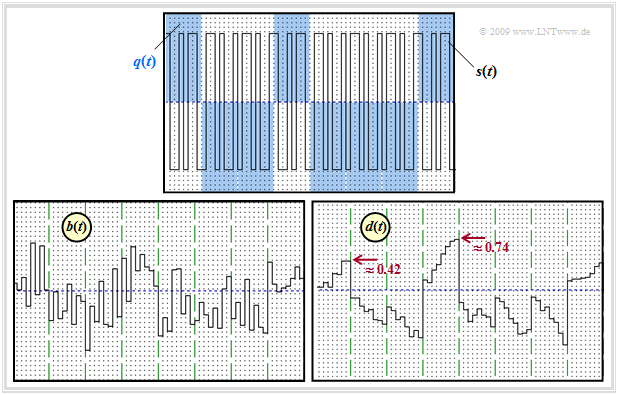## Block diagram and equivalent low-pass model

One possibility for realizing a CDMA system is the so-called  »Direct-Sequence Spread Spectrum«  $\text{(DS–SS)}$ ,  which is explained here using the block diagram.  The corresponding model in the equivalent low-pass range is shown below.Block diagram and equivalent low-pass model of direct-sequence spread spectrum

In both models,

• the distortion-free channel  $($AWGN and possibly interference from other users,  but no  intersymbol interference$)$  is highlighted in yellow,
• the  optimal receiver  $($matched filter plus threshold decider$)$ is highlighted in green.

Note:

In in the equivalent low-pass model the multiplications with the transmitter-side carrier signal  $z(t)$  and the receiverer-side carrier signal   $z_{\rm E}(t) =2\cdot z(t)$  are omitted.

$\text{This system can be characterized as follows:}$

• If the multiplication with the spreading signal  $c(t)$  at transmitter and receiver is omitted,  the result is a conventional  BPSK system  with the carrier  $z(t)$  and AWGN noise, characterized by the additive Gaussian noise signal  $n(t)$.  The second interference component  (interference from other participants)  is omitted:   $i(t) = 0$.
• For the following it is assumed  $($this is essential for direct-sequence spread spectrum!$)$ that the source signal  $q(t)$  has a rectangular NRZ curve.  Then the matched filter can be replaced by an integrator over a symbol duration  $T$    ⇒    "Integrate & Dump".  This is followed by the threshold decider.

## Principle and properties of band spreading methods

In the following we consider  Pseudo-Noise Band Spreading  in the equivalent low-pass region.  So the model outlined below applies.Low-pass model of direct-sequence spread spectrum
• Characteristic for this type of modulation is the multiplication of the bipolar and rectangular digital signal  $q(t)$  with a pseudo-random  $±1$ spreading sequence  $c(t)$:
$$s(t) = q(t) \cdot c(t) \hspace{0.05cm}.$$
• The duration  $T_c$  of a spreading chip is smaller than the duration  $T$  of a source symbol by the integer spreading factor  $J$,  so that the transmitted signal spectrum
$$S(f) = Q(f) \star C(f)$$
is wider than the spectrum  $Q(f)$ by approximately this factor  $J$.

$\text{In this context, please note in particular:}$

• In previous chapters,  a major goal of modulation has always been to be as bandwidth-efficient as possible.
• Here, in contrast,  we try to spread the signal over as wide a bandwidth as possible.
• The bandwidth expansion by  $J$  is necessary to allow several subscribers to use the same frequency band simultaneously.
• Ideally,  $2^J$  suitable spreading sequences can be found.  This makes a CDMA system for  $2^J$  simultaneous users feasible.

• An additional low-rate  "DS–SS signal"  can be transmitted over a frequency band that is otherwise used by FDMA channels with a higher data rate without significantly disrupting the main applications.  The band spread signal virtually disappears under the noise level of these signals.
• Targeted narrowband interferers  ("sinusoidal interferers")  can be combated well with this technique.  This military point of view was also decisive for the invention and further development of band spreading techniques.
• Furthermore,  the band spreading technique in general,  but especially  frequency hopping  $($fast discrete change of the carrier frequency over a wide range$)$  and  chirp modulation  $($continuous change of the carrier frequency during a bit interval$)$  also offer the possibility of better transmission over frequency-selective channels.

## Signal curves with a single participant

A disadvantage of direct-sequence spread spectrum modulation is that under unfavorable conditions interference can occur between the subscriber under consideration and other subscribers.

• This case is taken into account in the model by the interference quantity  $i(t)$.
• We initially consider only one transmitter, so that  $i(t) = 0$  is to be set.Signals of direct-sequence spread spectrum modulation in the noise-free case

$\text{Example 1:}$  The graph shows

• above the source signal  $q(t)$  – marked by blue background – and the band spread signal  $s(t)$  as solid black line,
• at the bottom left the signal  $b(t)$  after band compression,
• bottom right the detection signal  $d(t)$  after the integrator, directly before the decision maker.

Further notes:

1.   Discrete-time and normalized signal representation with rectangles spaced by the chip duration  $T_c$  is chosen.
2.   The spreading factor is  $J = 8$.
3.   As spreading sequence the  Walsh function no. 7  is used.
4.   All images show the noise-free case   ⇒   $n(t) = 0$.

To the individual signal curves is to be noted:

• The  $±1$ data signal  $q(t)$  is marked by the blue background.  After multiplication with the spreading signal  $c(t)$,  the result is the transmitted signal  $s(t)$  which is higher in frequency by the factor  $J = 8$.
• The spreading signal  $c(t)$  is periodic with  $T = J · T_c$  and thus has a line spectrum.  In the data bits  $1,\ 4,\ 8$:   $s(t)=c(t)$,  other times,  $s(t) = - c(t)$.
• After band compression at the receiver, i.e.,  after chipsynchronous multiplication by  $c(t) ∈ \{±1\}$   ⇒   $c^2(t) = 1,$  the signal  $b(t)$  is obtained.
• In the distortion-free and noise-free case:
$$b(t) = r(t) \cdot c(t) = s(t) \cdot c(t) = \big [ q(t) \cdot c(t) \big ] \cdot c(t) = q(t) \hspace{0.05cm}.$$
• Integrating  $b(t)$  over one bit at a time yields a linearly increasing or linearly decreasing signal  $d(t)$.  The step curve in the right image is solely due to the discrete-time representation.
• At the equidistant detection times the  $ν$–th amplitude coefficients  $a_ν$  of the source signal  $q(t)$ are valid in the distortion-free and noise-free case:
$$d (\nu T) = \frac{1}{T} \cdot \hspace{-0.1cm} \int_{(\nu -1 )T }^{\nu T}\hspace{-0.3cm} b (t )\hspace{0.1cm} {\rm d}t = a_\nu \in \{ +1, -1 \}\hspace{0.05cm}.$$Signals of direct-sequence spread spectrum modulation for  $10 · \lg \ (E_{\rm B}/N_0) = 6 \ {\rm dB}$

$\text{Example 2:}$  The two lower graphs change significantly from the first example when AWGN noise is considered.

The AWGN parameter is  $10 · \lg \ (E_{\rm B}/N_0) = 6 \ \rm dB$.    Then

• the band compressed signal  $b(t)$  is no longer sectionally constant, and
• the detection signal  $d(t)$  is no longer linearly increasing or decreasing.

After thresholding the samples  $d(νT)$,  one nevertheless obtains mostly the sought amplitude coefficients.  The vague statement "mostly" is quantifiable by the bit error probability  $p_{\rm B}$.   As

$$b(t) = \big [ s(t) + n(t) \big ] \cdot c(t) = q(t) + n(t) \cdot c(t)$$

and due to the fact that the statistical properties of white noise  $n(t)$  are not changed by the multiplication with the  $±1$ signal  $c(t)$,  the same result is obtained again as for the  conventional BPSK  without band spreading/band compression, independent of the spreading degree  $J$ :

$$p_{\rm B} = {\rm Q} \left( \hspace{-0.05cm} \sqrt { {2 \cdot E_{\rm B} }/{N_{\rm 0} } } \hspace{0.05cm} \right ) \hspace{0.05cm}.$$

## Additional sinusoidal interferer around the carrier frequency

We continue to assume only one participant.  In contrast to the calculation in the last section,  however,  there are now

• in addition to the AWGN noise  $n(t)$  also
• a narrowband interferer  $i(t)$  around the frequency  $f_{\rm I}$  with power  $P_{\rm I}$  and bandwidth  $B_{\rm I}$.

In the limiting case  $B_{\rm I} → 0$  the power-spectral density of this  "sinusoidal interferer"  is:

$${\it \Phi}_{\rm I}(f) = {P_{\rm I}}/{2} \cdot \big[ \delta ( f - f_{\rm I}) + \delta ( f + f_{\rm I}) \big ] \hspace{0.05cm}.$$

In a conventional transmission system without band spreading/band compression,  such a narrowband interferer would increase the error probability to an unacceptable extent.  In a system with band spreading   ⇒   "direct-sequence spread spectrum modulation",  the interfering influence is significantly lower,  since

• band compression acts as band spreading at the receiver with respect to the sinusoidal interferer,
• thus its power is distributed over a very wide frequency band  $B_c = 1/T_c \gg B$,
• the additional interfering power density in the useful frequency band  $(±B)$  is rather low and can be taken into account by a slight increase of AWGN noise power density  $N_0$.

With  $T = J · T_c$  and  $B = 1/T$  one obtains:

$$p_{\rm B} \approx {\rm Q} \left( \hspace{-0.05cm} \sqrt { \frac{2 \cdot E_{\rm B}}{N_{\rm 0} +P_{\rm I} \cdot T_c} } \hspace{0.05cm} \right ) = {\rm Q} \left( \hspace{-0.05cm} \sqrt { \frac{2 \cdot E_{\rm B}}{N_{\rm 0} } \cdot \left( \frac{1}{1+ P_{\rm I} \cdot T_c/N_0}\right ) } \hspace{0.05cm} \right )\hspace{0.3cm}\Rightarrow \hspace{0.3cm}\text{SNR degradation:} \ \frac{1}{\big[1 + P_{\rm I}/(J · N_0 · B)\big]}\hspace{0.05cm}.$$

The larger the spreading factor  $J$,  the smaller the increase in noise power due to the sinusoidal interferer.

Note:   This fact has led to the spreading factor  $J$  being often referred to  "spreading gain"  in the literature,  compare for example  [ZP85].

• These books are mostly about military applications of the band spreading methods.
• Sometimes the  "most favorable interferer"  is mentioned,  namely when the degradation is the largest.
• However,  we do not want to deal with such applications here.

But the above error probability equation can also be applied approximately when an unspread transmission of higher data rate and a spread spectrum system of lower rate operate in the same frequency band:   The interfering influence of the former system with bandwidth  $B_{\rm I}$  on the  spread spectrum system  can be treated approximately as a  "narrowband interferer"  as long as  $B_{\rm I}$  is sufficiently small.

$\text{Conclusions:}$

• With AWGN noise  (and also many other channels),  the bit error probability cannot be reduced by band spreading.
• In the best case,  band spreading results in the same bit error probability as BPSK  (without spreading).
• For our purposes,  band spreading is a necessary measure to be able to supply several subscribers simultaneously in the same frequency band.
• In the following,  we will only consider the CDMA aspect and therefore continue to speak of the spreading factor  $J$  and not of a  "spreading gain".